# Describing and Evaluating Models in DataRobot

September 13, 2021
by

This post was originally part of the DataRobot Community. Visit now to browse discussions and ask questions about DataRobot, AI Platform, data science, and more.

This article explains model evaluation techniques, including Lift ChartROC CurvePrediction Distribution graphs, and the Profit Curve tool. These are all calculated after Quick or full Autopilot has finished running on the data.

### Lift Chart

You can find the Lift Chart under the Evaluate > Lift Chart tab of the model you are interested in.  On the Y-axis you have the average target value, while on the X-axis you have the prediction distribution ranked and binned from lowest to highest. The blue and orange lines depict the average predicted and average real probability (respectively) for a particular bin.

If the model is predicting well, then the blue and orange lines should track one another.  This shows that the average of the predicted values should be similar to the average of the actuals for each bin. Below the the chart you can find tools for changing the partition that you are viewing, the number of bins on the X-axis, or the sorting order, or for exporting the data as a CSV format file or image.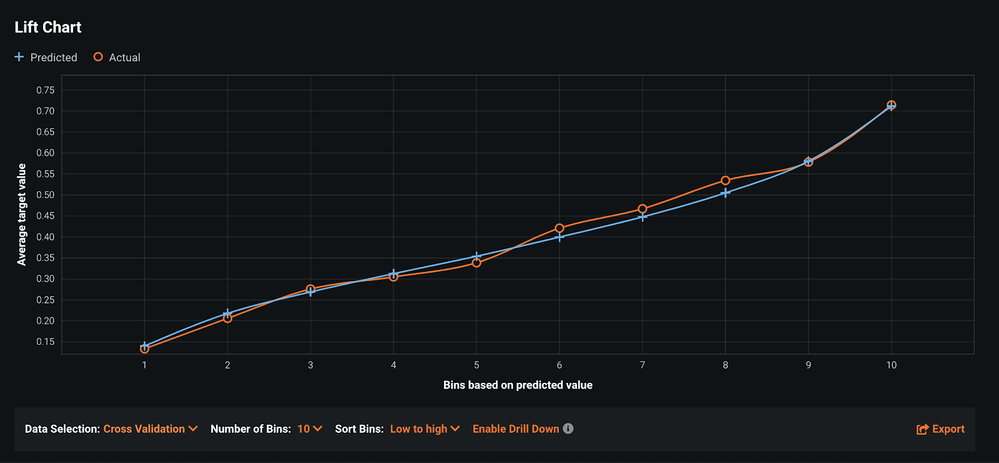Figure 1. Lift Chart

### ROC Curve

The ROC Curve tab (from the Evaluate tab) has a variety of tools typically used in evaluating models. This is only available for binary classification projects.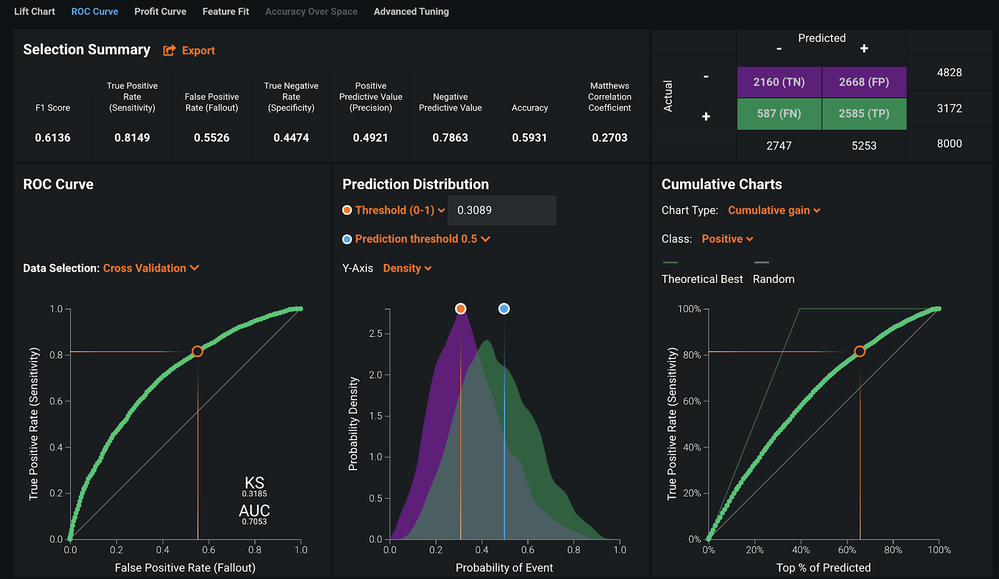Figure 2. ROC Curve

### Confusion Matrix

The Confusion Matrix tells you how each row of data was categorized for a given partition. You can change the partition on the bottom left of the overall ROC Curve tab.

Specifically this tells you how many rows ended up being True Negatives (correct rejections), False Positives (false alarms), False Negatives (misses), and True Positives (hits).  This is especially important to look at if you have a highly imbalanced target.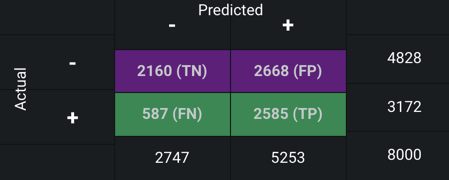Figure 3. Confusion Matrix

#### ROC Curve

The ROC Curve tells you the rate of True Positive (hits) vs. the False Positive (false alarms). There is typically a relationship between True Positives (hits) and False Positives (false alarms).  As you change the threshold you will be able to visualize this on the ROC Curve.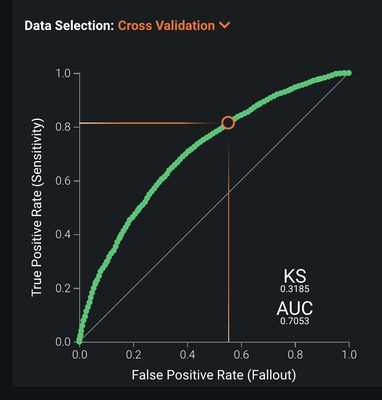Figure 4. ROC Curve

#### Prediction Distribution

The Prediction Distribution allows you to see the separation between the binary classes of the target. The purple distribution represents one class and the green distribution represents the other. You can see two threshold options. The display threshold (indicated with an orange dot) is designed to allow you to try out different thresholds and examine the impact on the confusion matrix and associated metrics. This threshold is automatically set to optimize the F1 score. The second threshold, the actual prediction threshold, is set to 0.5 by default and is being used by the model. If you find a better threshold by using the adjustable too in the graph, then you can replace that default by clicking on the dropdown.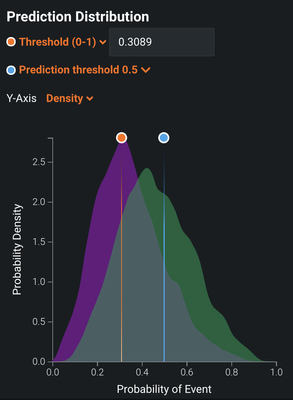Figure 5. Prediction Distribution

### Profit Curve

The Profit Curve tool (from the Evaluate tab) shows you what the profit would be with the current display threshold selected.  This assumes that all outcomes (True Positives, False Positives, True Negatives, and False Negatives) are worth the same amount of money. You can customize this by adding a new Payoff Matrix with custom values for your specific business problem. This is especially useful in scenarios where some outcomes are more costly than others.  In use cases like Fraud, for example, if you get a False Positive and check on a transaction that turns out to be valid, that is not a huge cost. However, if you get a False Negative and miss an incident of fraud, that can cost a lot of money (e.g., 1000s of dollars). You can simulate these kinds of scenarios here.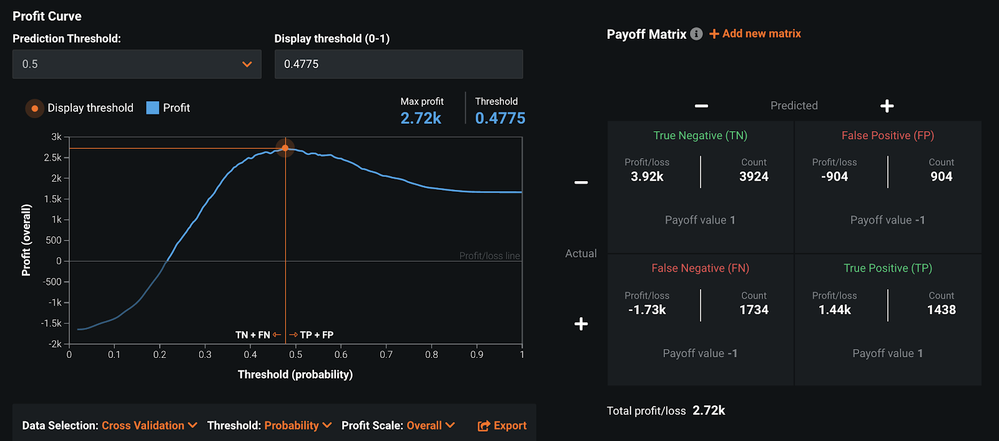Figure 6. Profit Curve

### Residuals

The Residuals plot (from the Evaluate tab) is used to evaluate regression models within a scatter plot. On the Y-axis you have the Predicted values and on the X-axis you have the Actuals. If these values overlap, or are very similar to one another, you will see them cluster around the diagonal line in the plot. In the image below you can see the values overlap quite well up to 40 and then start to diverge at the higher end of the distribution.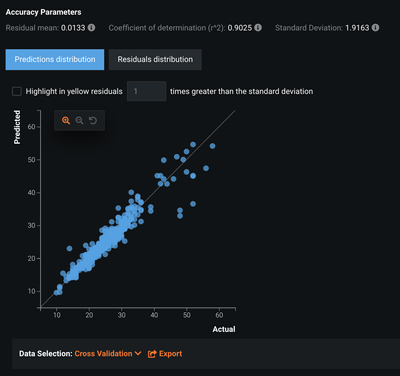Figure 7. Residuals

See the DataRobot public documentation: Lift ChartROC CurveProfit Curve, or Residuals.

Demo
See DataRobot in ActionLinda Haviland

Community Manager

Meet Linda Haviland
• Listen to the blog

Subscribe to DataRobot Blog
Thank you

We will contact you shortly

We’re almost there! These are the next steps:

• Look out for an email from DataRobot with a subject line: Your Subscription Confirmation.